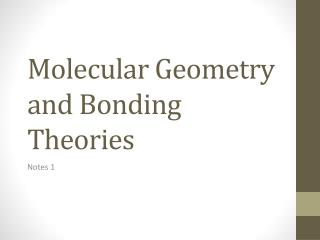DownloadDownload PresentationMolecular Geometry and Bonding Theories

# Molecular Geometry and Bonding Theories

Download Presentation## Molecular Geometry and Bonding Theories

- - - - - - - - - - - - - - - - - - - - - - - - - - - E N D - - - - - - - - - - - - - - - - - - - - - - - - - - -
##### Presentation Transcript

1. Molecular Shapes • Lewis Structures give atomic connectivity they tell what is physically connected to which atom but it tells little about geometry. • The shape of a molecule is determined by the bond angle • The angle made by the lines joining the nuclei of the atoms in a molecule • Consider CCl4 • Experimentally the bond angles are all 90o so the molecule is not planar all are located on vertices of a tetrahedron with C at the center • Geometries are such that they minimize electron repulsion

2. VSEPR • A covalent bond forms when two atoms electrons occupy the space between the nuclei • The region of sharing is an electron domain • A non bonding pair or lone pair defines an electron domain located principally on one atom • Example NH3 has three bonding pairs and one lone pair • VSEPR predicts geometries to minimize electron repulsions • The arrangement of electron domains about the central atom of an Abn molecule is its electron- domain geometry.

3. Electron domain geometries • Linear- two electron domains • Trigonal planar- three domains • Tetrahedral- four domains • Trigonalbipyramidal- five domains • Octahedral- six domains

4. Steps to geometry • 1. Draw the lewis structure • 2. count the total number of electron pairs around the central atom • 3. Arrange the electron pairs in one of the previous geometries to minimize the electron repulstions • 4. Determine the 3D structure of a molecules • 5. Ignore lone pairs in the molecular geometry • Describe the molecular geometry in terms of the angular arrangement of the bonded atoms • Multiple bonds count as one electron domain

5. The effect of Nonbonding electrons and multiple bonds on bond angle • We refine VSEPR to predict and explain slight distortions from ideal geometries • Consider three molecules with tetrahedral electron domain geometries CH4, NH3 and H2O. • By experiment HXH bond angle decreases from C (109.5o in CH4) to N (107 in NH3 to O(104.5 in H2O) • A bonding pair of electrons is attracted by two nuclei. They do not repel as much as the nonbonding pairs, which are primarily attracted to only one nucleus • Electron domains for nonbonding electron pairs thus exert greater repulsive forces on adjacent electron domains • They tend to compress bond angles • The bond angle decreases as the number of nonbonding electron pair increases.

6. Similarly,electrons in multiple bonds repel more than electrons in single bonds

7. Molecular shapes • Three atoms • Linear • Bent • Four atoms • Trigonal planar • Trigonalbipyramidal • T- shaped • Five atoms • Tetrahedral • Square planar • See Saw

8. Six atoms • Trigonalbipyramidal • Square pyramidal • Seven atoms • Octahedral